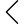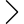34 products found
• s
• m
• l
• xl
• s
• m
• l
• xl
• s
• m
• l
• xl
• s
• m
• l
• xl
DOWN KANGAROO PATCHED HOODY
\$379.00 \$189.00
• s
• m
• l
• xl
DOWN KANGAROO PATCHED HOODY
\$379.00 \$189.00
• s
• m
• l
• xl
• 2xl
• s
• m
• l
• xl
• s
• m
• l
• xl
• s
• m
• l
• xl
• s
• m
• l
• xl
• s
• m
• l
• xl
• s
• m
• l
• xl
• s
• m
• l
• xl
• s
• m
• l
• xl
• s
• m
• l
• xl
• s
• m
• l
• xl
• s
• m
• l
• xl
• s
• m
• l
• xl
• s
• m
• l
• xl
• s
• m
• l
• xl
• s
• m
• l
• xl
• s
• m
• l
• xl
• s
• m
• l
• xl
• s
• m
• l
• xl
• s
• m
• l
• xl
• s
• m
• l
• xl
• s
• m
• l
• xl
• s
• m
• l
• xl
• s
• m
• l
• xl
• s
• m
• l
• xl
• s
• m
• l
• xl
• s
• m
• l
• xl
GEO-CUT LOUNGE PANTS - DYED
\$249.00 \$124.00
• s
• m
• l
• xl
GEO-CUT LOUNGE PANTS - DYED
\$249.00 \$124.00
• s
• m
• l
• xl
GEO-CUT LOUNGE PANTS - DYED
\$249.00 \$124.00
••